# SQL Server聚合函数与聚合开窗函数 （转载）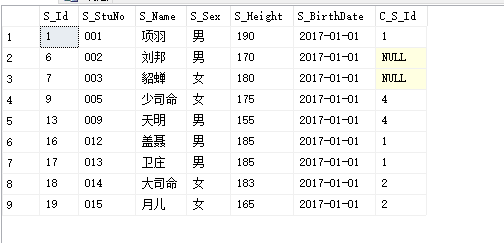COUNT（统计函数）：COUNT函数可以用来返回所有选中行的总行数，它有一个参数，可以是 ‘*’（即所有列）、’1’（效果同前者类似）和具体的列名。

```1     select count(1) from Student    -- 返回 9
2
3     select count(S_Sex) from Student    -- 返回 9
4
5     select count(distinct S_Sex) from Student    -- 返回 2，即去重之后性别只有两种```

COUNT_BIG（统计函数）：COUNT_BIG函数的使用方法和COUNT函数相同，只不过返回值为 bigint 数据类型。

SUM（求和函数）：SUM函数用于求和，计算指定列中所有不为空（null）的总和。返回值类型为： int

```1 --求所有学员的身高总和,因为字段类型是 varchar 类型，所以需要转换一下
2     select SUM(convert(int,S_Height)) from Student    -- 返回 1588
3
4     --求不同身高的学员的身高总和（去掉重复项）
5     select SUM(distinct convert(int,S_Height)) from Student -- 返回 1403```

AVG（求平均值函数）：AVG函数用于求平均值，计算指定列中所有不为空（null）的平均值。返回值类型为： int

```1     --求所有学员的平均身高,因为字段类型是 varchar 类型，所以需要转换一下
2     select AVG(convert(int,S_Height)) from Student    -- 返回 176
3
4     --求不同身高的学员的平均身高（去掉重复项）
5     select AVG(distinct convert(int,S_Height)) from Student -- 返回 175
6
7     --求男同学的平均身高
8     select AVG(convert(int,S_Height)) from Student where S_Sex='男'    -- 返回 177```

MAX（最大值函数）：MAX函数用于计算最大值，可以使用于numeric、char、varchar、money、smallmoney、或datetime列，但不能用于bit列。返回值类型为： int

```1     --求所有学员的最大身高,因为字段类型是 varchar 类型，所以需要转换一下
2     select MAX(convert(int,S_Height)) from Student    -- 返回 190
3
4     --求女同学的最大身高
5     select MAX(convert(int,S_Height)) from Student where S_Sex='女'    -- 返回 183```

MIN（最小值函数）：MIN函数用于计算最小值，可以使用于numeric、char、varchar、money、smallmoney、或datetime列，但不能用于bit列。返回值类型为： int

```1     --求所有学员的最小身高,因为字段类型是 varchar 类型，所以需要转换一下
2     select MIN(convert(int,S_Height)) from Student    -- 返回 155
3
4     --求女同学的最小身高
5     select MIN(convert(int,S_Height)) from Student where S_Sex='女'    -- 返回 165```

STDEV（标准偏差值函数）：STDEV函数用于计算标准偏差值，即每一个数值与平均值的标准差。返回值类型为：float

```1     --求所有学员的身高标准差,因为字段类型是 varchar 类型，所以需要转换一下
2     select STDEV(convert(int,S_Height)) from Student    -- 返回 11.2928197443234
3
4     --求女同学的身高标准差
5     select STDEV(convert(int,S_Height)) from Student where S_Sex='女'    -- 返回 7.88986691902975```

VAR（方差值函数）：VAR函数用于计算方差值。返回值类型为：float

```1     --求所有学员的身高方差,因为字段类型是 varchar 类型，所以需要转换一下
2     select VAR(convert(int,S_Height)) from Student    -- 返回 127.527777777781
3
4     --求女同学的身高方差
5     select VAR(convert(int,S_Height)) from Student where S_Sex='女'    -- 返回 62.25```

HAVING：HAVING子句仅用于带有 GROUP BY 子句的查询语句中，WHERE子句用于每一行数据的筛选（在变成一个组的某一部分之前），而HAVING子句用于分组的聚合值的筛选。

```1 --查询身高至少有一个相同的值
2     select S_Height,count(S_Height)
3     from Student
4     group by S_Height
5     having count(1)>1```

```1 　　select S_StuNo,S_Name,S_Height,
2     sum(convert(int,S_Height)) over(order by S_Height)
3     from Student```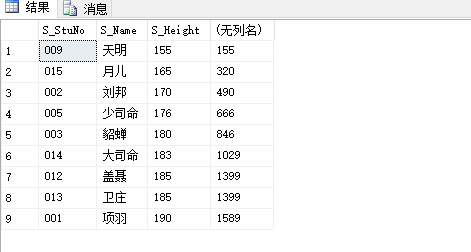```1 　　select S_StuNo,S_Name,S_Height,
2     sum(convert(int,S_Height)) over(partition by S_Height order by S_Height)
3     from Student```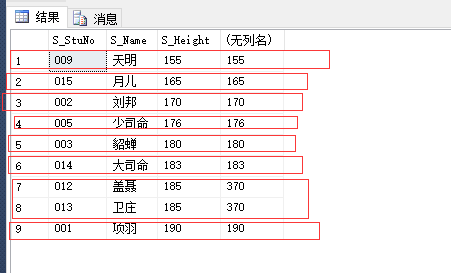```1     select S_StuNo,S_Name,S_Height,
2     count(S_Height) over(order by S_Height)
3     from Student```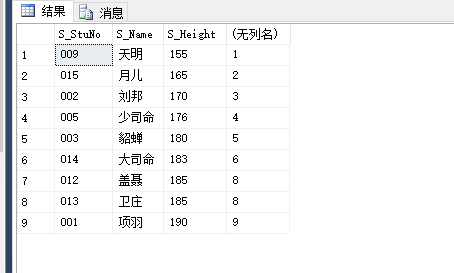```1     select S_StuNo,S_Name,S_Height,
2     count(S_Height) over(partition by S_Height order by S_Height)
3     from Student```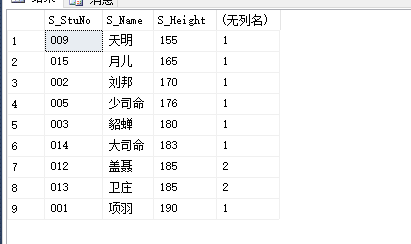```1 　　select S_StuNo,S_Name,S_Height,
2     AVG(convert(int,S_Height)) over(order by S_Height)
3     from Student```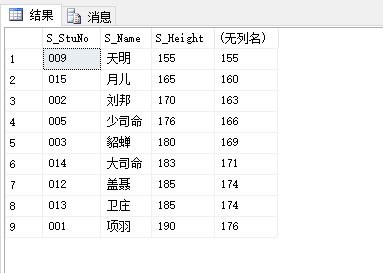```1 　　select S_StuNo,S_Name,S_Height,
2     AVG(convert(int,S_Height)) over(partition by S_Height order by S_Height)
3     from Student```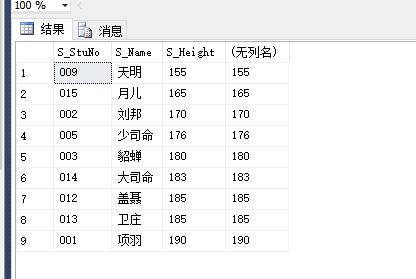https://www.cnblogs.com/Brambling/p/6711937.html